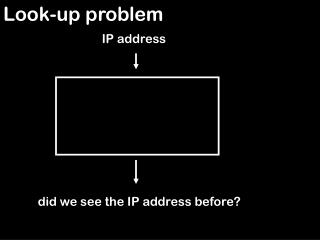DownloadDownload PresentationLook-up problem

# Look-up problem

Télécharger la présentation## Look-up problem

- - - - - - - - - - - - - - - - - - - - - - - - - - - E N D - - - - - - - - - - - - - - - - - - - - - - - - - - -
##### Presentation Transcript

1. Look-up problem IP address did we see the IP address before?

2. Hashing + chaining use IP address as an index linked list index hash function IP address

3. How to choose a hash function? depends on the distribution of the data x [0,1] x x.n interpret x as a number x  x mod n IP-addresses n=256 bad n=257 good?

4. Universal hash functions choose a hash function “randomly” n = number of entries in the hash table U = the universe h: U  {0,...,n-1} a hash function

5. Universal hash functions choose a hash function “randomly” n = number of entries in the hash table U = the universe h: U  {0,...,n-1} a hash function a set of hash functions H is universal if x,y U and random h  H P ( h(x) = h(y) )  1/n

6. Universal hash functions a set of hash functions H is universal if x,y U and random h  H P ( h(x) = h(y) )  1/n For IP addresses choose a1,a2,a3,a4  {0,1,...,256} (x1,x2,x3,x4)  a1x1+a2x2+a3x3+a4x4 mod 257

7. Perfect hashing Goal: worst-case O(1) search space used O(m) static set of elements

8. Perfect hashing Goal: worst-case O(1) search space used O(m) static set of elements n = m2 i.e., space used (m2) H = family of universal hash functions hash function h H with no collision

9. Perfect hashing Goal: worst-case O(1) search space used O(m) n = m H = family of universal hash functions x1,...,xn the number of elements that map to 1,2,...,n h H such that xi2 = O(m)

10. h H such that xi2 = O(m) Perfect hashing Goal: worst-case O(1) search space used O(m) n = m H = family of universal hash functions x1,...,xn the number of elements that map to 1,2,...,n secondary hash table of size xi2

11. Bloom filter Goal: store an m element subset of IP addresses IP address HASH HASH HASH 0 0 0 n-bits of storage

12. Bloom filter - insert INSERT(x) for i from 1 to k do A(hi(x))  1 IP address HASH HASH HASH 1 1 1 n-bits of storage

13. Bloom filter – member MEMBER(x) for i from 1 to k do if A(hi(x))=0 then return FALSE return TRUE IP address HASH HASH HASH 1 1 1 n-bits of storage

14. Bloom filter – member MEMBER(x) for i from 1 to k do if A(hi(x))=0 then return FALSE return TRUE sometimes gives false positive answer error parameter: false positive probability

15. Bloom filter – analysis error parameter: false positive probability m = number of items to be stored n = number of bits of storage k = number of hash functions

16. Bloom filter – analysis error parameter: false positive probability m = number of items to be stored n = number of bits of storage k = number of hash functions p = fraction of the bits filled p  e-km/n

17. Bloom filter – analysis error parameter: false positive probability m = number of items to be stored n = number of bits of storage k = number of hash functions p  e-km/n p = fraction of the bits filled false positive probability (1-p)k

18. Bloom filter – analysis error parameter: false positive probability m = number of items to be stored n = number of bits of storage k = number of hash functions optimal k  0.7 m/n false positive rate  0.6185m/n